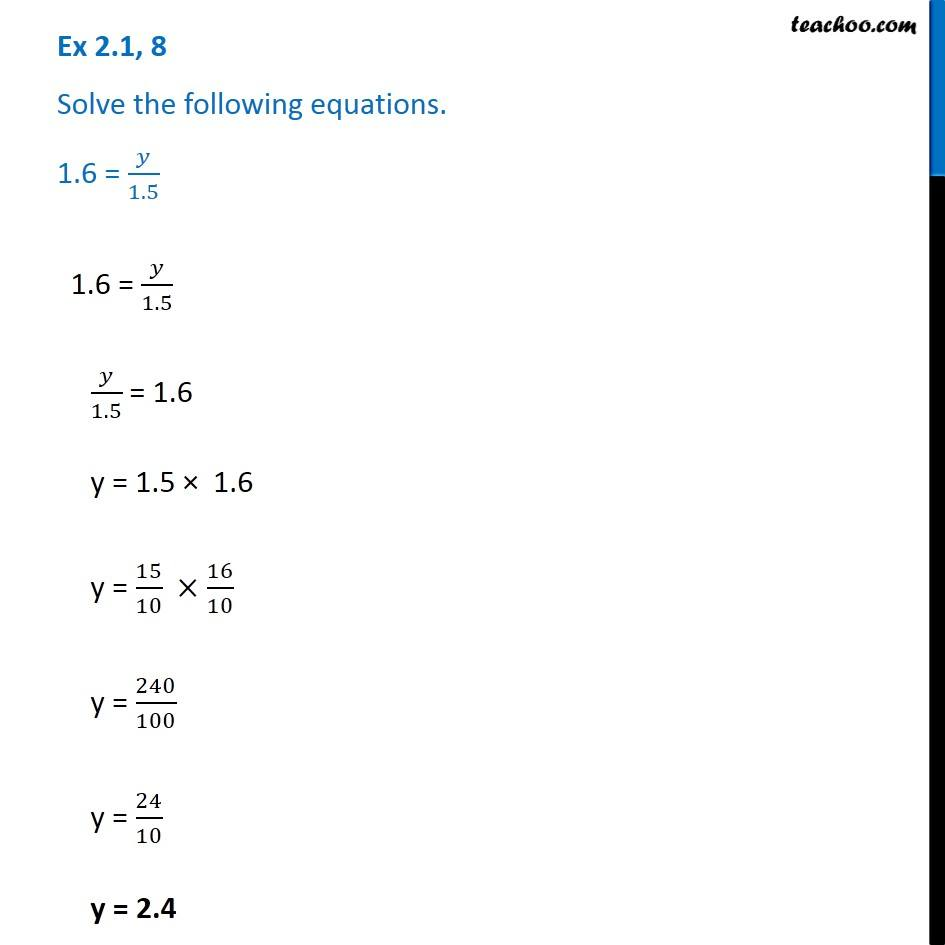Solving easy equations

Chapter 2 Class 8 Linear Equations in One Variable
Serial order wiseLearn in your speed, with individual attention - Teachoo Maths 1-on-1 Class

### Transcript

Question 8 - Chapter 2 Class 8 NCERT - Linear Equations in one variable Solve the following equations. 1.6 = y/1.5 1.6 = y/1.5 y/1.5 = 1.6 y = 1.5 × 1.6 y = 15/10 × 16/10 y = 240/100 y = 24/10 y = 2.4